Technical Mock Test CE (SSC JE)- 2

# Technical Mock Test CE (SSC JE)- 2

Test Description

## 100 Questions MCQ Test Civil Engineering SSC JE (Technical) | Technical Mock Test CE (SSC JE)- 2

Technical Mock Test CE (SSC JE)- 2 for Civil Engineering (CE) 2023 is part of Civil Engineering SSC JE (Technical) preparation. The Technical Mock Test CE (SSC JE)- 2 questions and answers have been prepared according to the Civil Engineering (CE) exam syllabus.The Technical Mock Test CE (SSC JE)- 2 MCQs are made for Civil Engineering (CE) 2023 Exam. Find important definitions, questions, notes, meanings, examples, exercises, MCQs and online tests for Technical Mock Test CE (SSC JE)- 2 below.
Solutions of Technical Mock Test CE (SSC JE)- 2 questions in English are available as part of our Civil Engineering SSC JE (Technical) for Civil Engineering (CE) & Technical Mock Test CE (SSC JE)- 2 solutions in Hindi for Civil Engineering SSC JE (Technical) course. Download more important topics, notes, lectures and mock test series for Civil Engineering (CE) Exam by signing up for free. Attempt Technical Mock Test CE (SSC JE)- 2 | 100 questions in 100 minutes | Mock test for Civil Engineering (CE) preparation | Free important questions MCQ to study Civil Engineering SSC JE (Technical) for Civil Engineering (CE) Exam | Download free PDF with solutions
 1 Crore+ students have signed up on EduRev. Have you?
Technical Mock Test CE (SSC JE)- 2 - Question 1

### Match List-I with List-II and select the correct answer using the code given below the lists: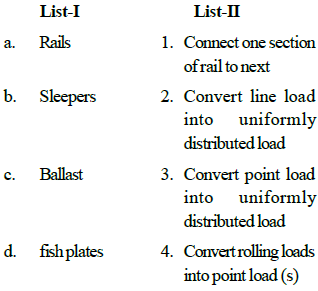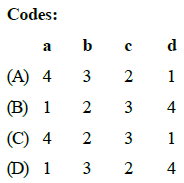Technical Mock Test CE (SSC JE)- 2 - Question 2

### A rail which is tapered to a toe at one end and has a heel at the other end in called as:

Technical Mock Test CE (SSC JE)- 2 - Question 3

### For a chord of 60 m, the mid-ordinate for a circular curve of 50 m radius will be

Technical Mock Test CE (SSC JE)- 2 - Question 4

The true length of a line is known to be 200 m. when this is measured with a 20 m tape, the length is 200.80 m. The correct length of the 20 m tape is

Detailed Solution for Technical Mock Test CE (SSC JE)- 2 - Question 4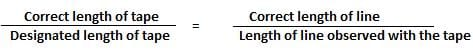Correct length of tape = 200 / 200.80 * 20 = 19.92 m

Technical Mock Test CE (SSC JE)- 2 - Question 5

Consider the following assumptions of Bowditch method:
1. Angular measurements are more precise than linear measurements.
2. Linear measurements are more precise than angular measurements.
3. Errors in linear measurements are proportional to√ L .
4. Correction to latitude or departure of any side
= Total error in L (or D)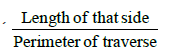Technical Mock Test CE (SSC JE)- 2 - Question 6

The arithmetical check for the computation of R.L. by "rise and fall" method is given by

Technical Mock Test CE (SSC JE)- 2 - Question 7

Match List-I with List-II and select the correct answer using the codes given below the lists: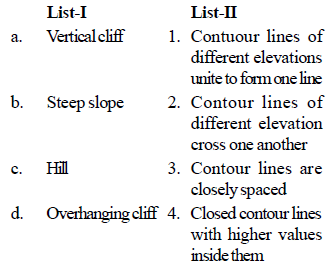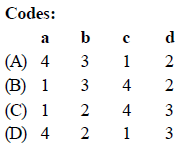Technical Mock Test CE (SSC JE)- 2 - Question 8

Match List-I with List-II and select the correct answer using the codes: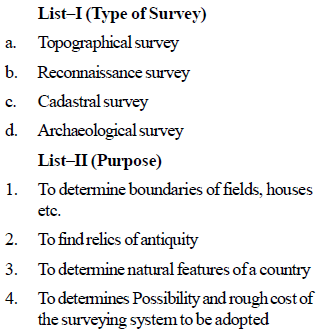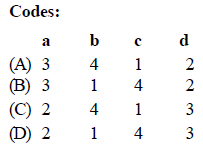Technical Mock Test CE (SSC JE)- 2 - Question 9

Which one of the following pairs is not correctly matched ?

Technical Mock Test CE (SSC JE)- 2 - Question 10

The dry density of a soil is 1.5 g/cc. If the saturation water content were 50% then its saturated density and submerged density would, respectively, be

Detailed Solution for Technical Mock Test CE (SSC JE)- 2 - Question 10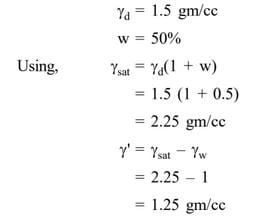Technical Mock Test CE (SSC JE)- 2 - Question 11

If s is the shear strength , c and f are shear strength parameters, and sn is the normal stress at failure , then Coulonb's equation for shear strength of the soil can be represented by

Technical Mock Test CE (SSC JE)- 2 - Question 12

Earth pressure and resultant possibilities of wall movement are shown in the below diagram. The point market X in the diagram denotes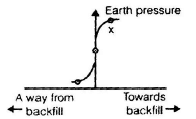Technical Mock Test CE (SSC JE)- 2 - Question 13

Given that for a soil deposit,

K0 = earth pressure coefficient at rest,

Ka = active earth pressure coefficient

Kp= passive earth pressure coefficient and m -Poisson's ratio, the value of (1 – m)/m is given by

Technical Mock Test CE (SSC JE)- 2 - Question 14

If D is the duration, ES and EF are the earliest start and finish, LS and LF are latest start and latest finish time, then the following relation holds good

Technical Mock Test CE (SSC JE)- 2 - Question 15

Match List-I (Property)with List-II (Slope of the curve) and select the correct answer using
the code given below the lists: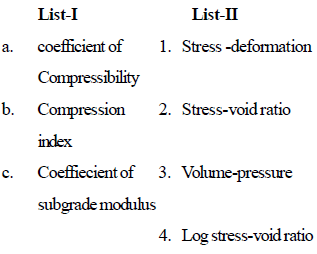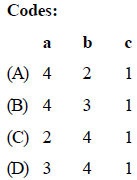Technical Mock Test CE (SSC JE)- 2 - Question 16

A part of the Newmark's influence chart with four concentric circles is shown in the belowfigure. If the hateched areas 1 and 2 are loaded separately with the same intensity of loading, then the intensity of pressure yielded.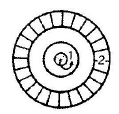Technical Mock Test CE (SSC JE)- 2 - Question 17

How many types of dressings are there with respect to the place of work?

Detailed Solution for Technical Mock Test CE (SSC JE)- 2 - Question 17

There are two types of dressings based on the place of work. They are quarry dressing and site dressing. Quarry dressing is carried out right after quarrying whereas site dressing is done after transporting the quarried rock to the site.

Technical Mock Test CE (SSC JE)- 2 - Question 18

Match List-I with List-II and select the correct answer using the code given below the lists: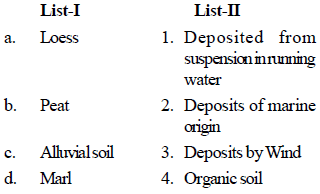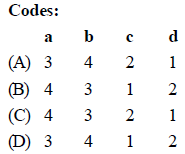Technical Mock Test CE (SSC JE)- 2 - Question 19

Match List-I (Soils) with List-II (Group symbols) and select the correct answer using the
code given below the lists: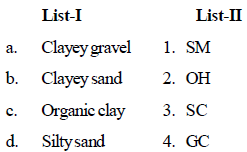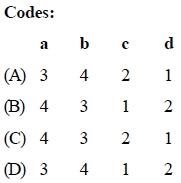Technical Mock Test CE (SSC JE)- 2 - Question 20

In the case of isolated square concrete footing, match the locations at which the stress resultants are to be checked, where d is effective depth of footing and select the correct answer using the codes given below the list :  Stress Resultant LocationA. Bending moment  1. At face of column B. One way shear 2. At d⁄ 2 from face of column C. Punching shear 3 At d from face of column?

Technical Mock Test CE (SSC JE)- 2 - Question 21

A river is the source of water for water supply to a town. Its water is very turbid and polluted. The correct sequence of steps for freating the river water would be for treating the river water would be

Detailed Solution for Technical Mock Test CE (SSC JE)- 2 - Question 21
• Coagulation and Flocculation

Coagulation and flocculation are often the first steps in water treatment. Chemicals with a positive charge are added to the water. The positive charge of these chemicals neutralizes the negative charge of dirt and other dissolved particles in the water. When this occurs, the particles bind with the chemicals and form larger particles, called floc.

• Sedimentation

During sedimentation, floc settles to the bottom of the water supply, due to its weight. This settling process is called sedimentation.

• Filtration

Once the floc has settled to the bottom of the water supply, the clear water on top will pass through filters of varying compositions (sand, gravel, and charcoal) and pore sizes, in order to remove dissolved particles, such as dust, parasites, bacteria, viruses, and chemicals.

• Disinfection

After the water has been filtered, a disinfectant (for example, chlorine, chloramine) may be added in order to kill any remaining parasites, bacteria, and viruses, and to protect the water from germs when it is piped to homes and businesses.

•

•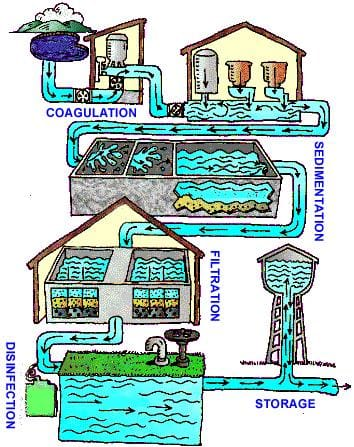Technical Mock Test CE (SSC JE)- 2 - Question 22

Uniformity coefficient of filter sand is given by

Technical Mock Test CE (SSC JE)- 2 - Question 23

Match List-I with List II ans select the correct answer using the codes given below the lists: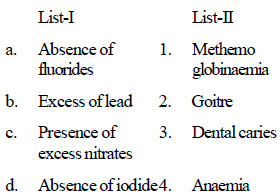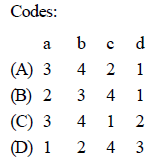Technical Mock Test CE (SSC JE)- 2 - Question 24

Which one of the following would help prevent the escape of foul sewer gases from a water closet ?

Technical Mock Test CE (SSC JE)- 2 - Question 25

Consider the following statements:

The daily per capital consumption of water apparently increases with

1. Higher standard of living of people

2. availability of sewerage in the city

3. metered water supply

4. wholesome and potable quality of water.

Which of these statement are correct?

Technical Mock Test CE (SSC JE)- 2 - Question 26

Which one of the following sewage treatement units has a parshall flume ?

Technical Mock Test CE (SSC JE)- 2 - Question 27

For fish habitat in a river, the minimum dissolved oxygen required is

Technical Mock Test CE (SSC JE)- 2 - Question 28

Wrought iron contains carbon upto

Technical Mock Test CE (SSC JE)- 2 - Question 29

Aerobic method of composting practised in India is called

Technical Mock Test CE (SSC JE)- 2 - Question 30

Which one of the following filters will produce water of higher bacteriological quality?

Technical Mock Test CE (SSC JE)- 2 - Question 31

Match List–I (Control structures) with List II (Functions of the Control structures) and select
the correct answer suing the codes given below the lists: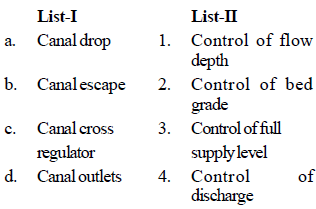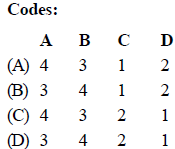Technical Mock Test CE (SSC JE)- 2 - Question 32

For medium silt whose average grain size is 0.16 mm, Lacey's silt factor is likely to be

Technical Mock Test CE (SSC JE)- 2 - Question 33

The total number of independent equations that form Lacey's regime theory is

Technical Mock Test CE (SSC JE)- 2 - Question 34

Consider the following:

Garret's diagram for the design of irrigationchannel is based on

1. Kennedy's theory

2. lacey's theory

3. Kutter's formula

4. Manning's formula

Which of these are correct ?

Technical Mock Test CE (SSC JE)- 2 - Question 35

Water present in an artesian aquifer is usually

Technical Mock Test CE (SSC JE)- 2 - Question 36

Match List–I (Name of scientist) with List II (Contribution of field of hydrology) and select
the correct answer suing the codes given below the lists: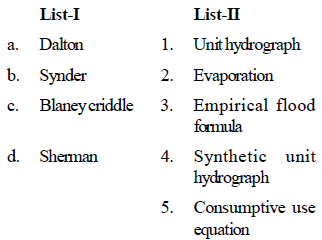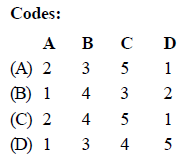Technical Mock Test CE (SSC JE)- 2 - Question 37

In a linear reservior , the

Technical Mock Test CE (SSC JE)- 2 - Question 38

Match List–I with List II and select the correct answer suing the codes given below the lists: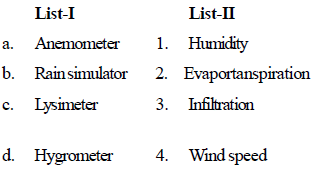Technical Mock Test CE (SSC JE)- 2 - Question 39

Match List–I with List II and select the correct answer suing the codes given below the lists: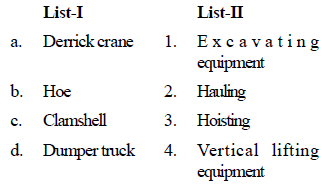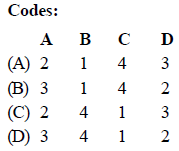Technical Mock Test CE (SSC JE)- 2 - Question 40

In the time-cost analysis, the cost slope is defined as

Technical Mock Test CE (SSC JE)- 2 - Question 41

Pay-back period gives an estimate of

Technical Mock Test CE (SSC JE)- 2 - Question 42

Give that

d = effective depth

b = width and

D = overall depth

the maximum area of compression reinforcementin a beam is

Technical Mock Test CE (SSC JE)- 2 - Question 43

The final deflection due to all loads including the effects of temperature, creep and shrinkage and measured from as cast level of supports of floors, roofs and all other horizontal members should NOT exceed

Technical Mock Test CE (SSC JE)- 2 - Question 44

A reinfirced concrete slab is 75 mm thick. The maximum size of reinforcement bar that can be used is

Detailed Solution for Technical Mock Test CE (SSC JE)- 2 - Question 44

As per IS:456–2000, Cl. 26.5.2.2, the diameter of reinforcing bars shall not exceed one-eight of the total thickness of the slab. Hence, maximum diameter for slab thickness of 75 mm should be 8 mm.

Technical Mock Test CE (SSC JE)- 2 - Question 45

Drops are provided in flats slabs to resist

Technical Mock Test CE (SSC JE)- 2 - Question 46

Consider the following statements:

Lug angles are used to

(1) increase the length of the end connectionsangle section

(2) decrease the length of end connections ofangle section

(3) increase the lengths of the end connectionsof channel section

(4) decrease the lengths of end connections ofchannel section

Which of the statements given above are correct?

Technical Mock Test CE (SSC JE)- 2 - Question 47

As per IS : 456-1978, the ratio of stress in concrete to its characteristic strength at collapse in flexure for design purposes is taken as

Detailed Solution for Technical Mock Test CE (SSC JE)- 2 - Question 47

The compressive strength of concrete in the structure shall be assumed to be 0.67 times the characteristics strength. The partial safety factor of 1.5 shall be applied in addition to this Thus stress in concrete at collapse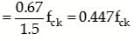Technical Mock Test CE (SSC JE)- 2 - Question 48

Lateral ties in RC columns are provided to resist?

Technical Mock Test CE (SSC JE)- 2 - Question 49

A bolt is subjected to a shear stress of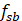and a tensile stress of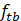. If the permissible stresses in shear and tension are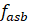and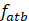respectively then the stress should satisfy:

Detailed Solution for Technical Mock Test CE (SSC JE)- 2 - Question 49

As per IS code no. 800:2007 for design of steel structure as per provision no.

11.6.2.5 If the bolt is subjected to combined shear and
tension, the actual shear and axial stresses calculated
then the
expression given below should satisfy: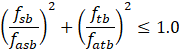where&= actual shear and tensile stresses
respectively, and&= permissible ‘hear and tensile ‘tresses respectively.

Technical Mock Test CE (SSC JE)- 2 - Question 50

Partial safety factor for concrete and steel are 1.5 and 1.15 respectively, because

Technical Mock Test CE (SSC JE)- 2 - Question 51

As compared to working stress method of design, limit state method takes concrete to

Technical Mock Test CE (SSC JE)- 2 - Question 52

Upper yield point in the stress-strain curve in structural steel can be avoided by

Technical Mock Test CE (SSC JE)- 2 - Question 53

The effective length of the fillet weld is

Technical Mock Test CE (SSC JE)- 2 - Question 54

Match List-I with List-II and select the correct answer using the codes given below the lists: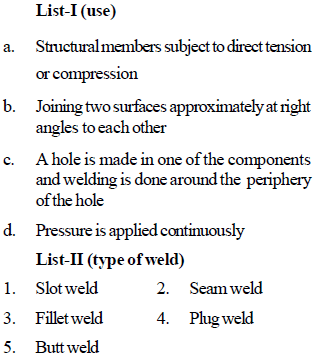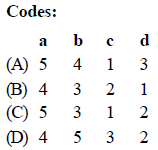Technical Mock Test CE (SSC JE)- 2 - Question 55

The economic spacing of a roof truss depends upon the

Technical Mock Test CE (SSC JE)- 2 - Question 56

Consider the following statements :

Bearing stiffeners are provided in a plate girder

1. to avoid local bending failure of flange.

2. to prevent buckling of web.

3. to strengthen the web.

Which of these statements are correct ?

Technical Mock Test CE (SSC JE)- 2 - Question 57

Match List-I (Propoerties) with List-II (Stress points labelled 1, 12, 3 and 4) and select the
correct answer using the codes given below the lists: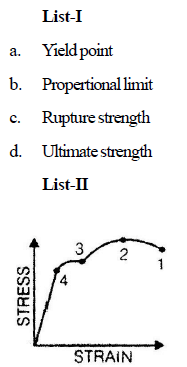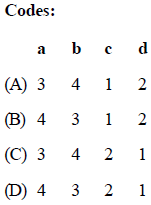Technical Mock Test CE (SSC JE)- 2 - Question 58

Which one of the following is the mode of failure in a fillet weld material?

Technical Mock Test CE (SSC JE)- 2 - Question 59

The maximum permissible slenderness ratio of a member carrying loads resulting from wind, is

Technical Mock Test CE (SSC JE)- 2 - Question 60

Match List-I (Failure Mode) with List-II (Reason) and select the correct answer using the
codes given below the lists: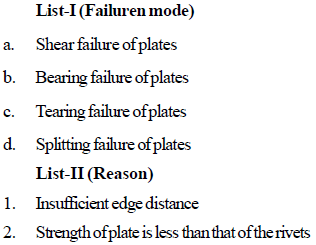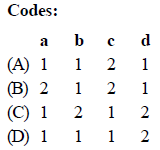Technical Mock Test CE (SSC JE)- 2 - Question 61

The working stress for structural steel in tension is of the order of

Technical Mock Test CE (SSC JE)- 2 - Question 62

Which one of the following is the chronological sequence is regard to road construction/developement ?

Technical Mock Test CE (SSC JE)- 2 - Question 63

Which of the criteria given below are used for the design of valley vertical curves on roads ?

1. Rider comfort.

3. Drainage

Selected the correct answer using the codes given below:

Technical Mock Test CE (SSC JE)- 2 - Question 64

Which one of the following pairs is NOT correctly matched ?

Technical Mock Test CE (SSC JE)- 2 - Question 65

In cement concrete pavements, tie bars are installed in

Technical Mock Test CE (SSC JE)- 2 - Question 66

For carrying out bituminous patch work during the rainy season, the most suitable binder is

Technical Mock Test CE (SSC JE)- 2 - Question 67

Match List-I with List-II and select the correct answer using the codes given below the lists: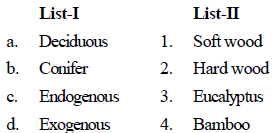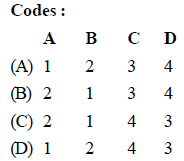Technical Mock Test CE (SSC JE)- 2 - Question 68

The modulus of elasticity of timber is about

Technical Mock Test CE (SSC JE)- 2 - Question 69

Which of the following pairs in respect of ordinary portland cement (OPC) are correctly matched ?

1. Initial setting time .......... 30 minutes

2. Final setting time ........ 10 hours

3. Normal consistency..... 10%

Select the correct answer using the codes givenbelow : Codes :

Technical Mock Test CE (SSC JE)- 2 - Question 70

High alumina cement is produced by fusing together a mixture of

Technical Mock Test CE (SSC JE)- 2 - Question 71

Which one of the following types of steels is used in the manufacture of rails?

Technical Mock Test CE (SSC JE)- 2 - Question 72

Linseed oil is used in paints as

Technical Mock Test CE (SSC JE)- 2 - Question 73

Before testing setting time of cement one should test for

Technical Mock Test CE (SSC JE)- 2 - Question 74

The tempeture range in a cement kiln is

Technical Mock Test CE (SSC JE)- 2 - Question 75

Match List–I (Admistures) with List II (Chemicals) and select the correct answer suing
the codes given below the lists: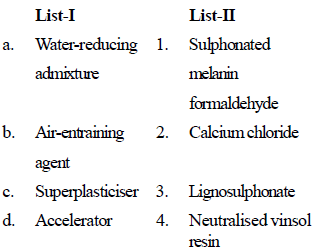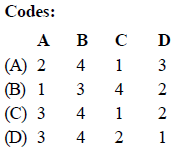Technical Mock Test CE (SSC JE)- 2 - Question 76

The deformation of a bar under its own weight as compared to that when subjected to a direct axial load equal to its own weight will be

Technical Mock Test CE (SSC JE)- 2 - Question 77

A solid uniform metal bar of diameter D and length L is hanging vertically from its upper end.The elongation of the bar due to self weight is

Technical Mock Test CE (SSC JE)- 2 - Question 78

If Poisson's ratio of a material is 0.5, then the elastic modulus for the material is

Technical Mock Test CE (SSC JE)- 2 - Question 79

The volumetric strain in case of a thin cylindrical shell of diameter 'd', thickness 't', subjected to internal pressure 'P' is

Technical Mock Test CE (SSC JE)- 2 - Question 80

Match List – I (State of stress) with List -II (kind of loading) and select the correct answer using the codes given below the lists: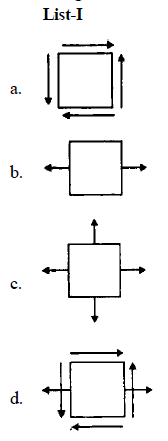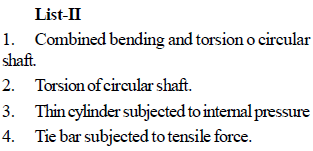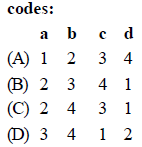Technical Mock Test CE (SSC JE)- 2 - Question 81

In a strained material, normal stresses on two mutually perpendicular planes are σx and σy (both alike) accompanied by a shear stress τxy. One of the principal stresses will be zero, only it

Detailed Solution for Technical Mock Test CE (SSC JE)- 2 - Question 81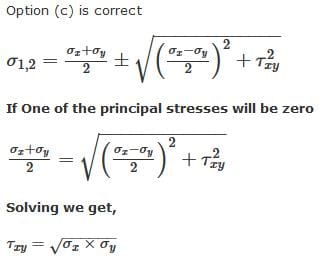Technical Mock Test CE (SSC JE)- 2 - Question 82

Auto frettage is the method of

Technical Mock Test CE (SSC JE)- 2 - Question 83

In a thick cylinder pressurized from inside, the hoop stress is maximum at

Technical Mock Test CE (SSC JE)- 2 - Question 84

A simply supported beam is loaded as shown is the figure below.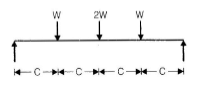The maximum shear force in the beam will be

Technical Mock Test CE (SSC JE)- 2 - Question 85

In a beam of circular cross-section, the shear stress variation across a cross section is

Technical Mock Test CE (SSC JE)- 2 - Question 86

The distribution of shear stress of a beam is shown in the given figure.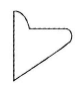Technical Mock Test CE (SSC JE)- 2 - Question 87

A shaft is subjected to torsion as shown in the figure below.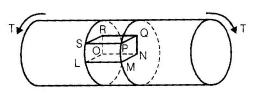Which of the following figures represents the shear stress on the element LMNOPQRS?

Technical Mock Test CE (SSC JE)- 2 - Question 88

A beam subjected to a load P is shown in the figure below.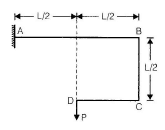The bending moment at the supports A of thebeam will be

Technical Mock Test CE (SSC JE)- 2 - Question 89

Match List-I (Rheological equation) with List- II (Types of fluids) and select the correct answer
using the codes given below the lists:
List I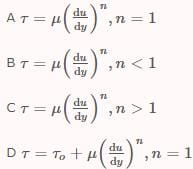List II

1. Bingham plastic
2. Dilatant fluid
3. Newtonian fluid
4. Pseudo-plastic fluid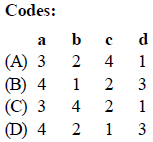Technical Mock Test CE (SSC JE)- 2 - Question 90

An oil of specific gravity 0.9 has viscosity of 0.28 stokes at 38ºC. What will be its viscosity in Ns/ m2?

Detailed Solution for Technical Mock Test CE (SSC JE)- 2 - Question 90

Concept:
Kinematic viscosity (ν) is defined as the ratio of dynamic viscosity (μ) to density (ρ) of fluid.
ν = μ/ρ
SI unit of kinematic viscosity is m2/s. CGS unit is stoke.
1 stoke = 1 cm2/s = 10-4 m2/s

Calculation:
Given: Specific gravity = ρ/ρwater = 0.9
ρ = 0.9 × 1000 kg/m3
ν = 0.28 stoke = 0.28 × 10-4 m2/s
ρ = 0.28 × {10^{ - 4}} × 0.9 × 1000 = 0.0252~ N.s/m^2

Technical Mock Test CE (SSC JE)- 2 - Question 91

The depth of fluid is measured in vertical zdirection; xand y are the other two directions and are mutually perpendicular. The static pressure variation in the fluid is given by (symbols have the usual meaning)

Technical Mock Test CE (SSC JE)- 2 - Question 92

A 70 kg person walds on snow with a total foot implint area of 500 cm2. What pressure does he exert on show?

Technical Mock Test CE (SSC JE)- 2 - Question 93

Manometer is a device used for measuring

Technical Mock Test CE (SSC JE)- 2 - Question 94

A rectangular water tank, full to the brim, has its length, breadth and height in the ratio of 2: 1 : 2. The ratio of hydrostatic forces at the bottom to that at any larger vertical surface is

Technical Mock Test CE (SSC JE)- 2 - Question 95

Three immiscible liquids of specific densities r, 2r and 3r are kept in a jar. The height of theliquied in the jar and at the piezometer fitted to the bottom of the jar are as shown in the figure below.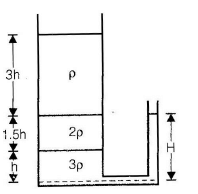The ratio H/h is .

Detailed Solution for Technical Mock Test CE (SSC JE)- 2 - Question 95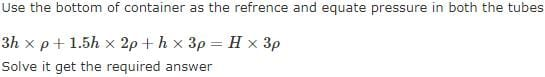Technical Mock Test CE (SSC JE)- 2 - Question 96

Technical Mock Test CE (SSC JE)- 2 - Question 97

The Euler's equation of motion is a statement of

Technical Mock Test CE (SSC JE)- 2 - Question 98

When is Bernoulli's equation applicable between any two points in a flow field?

Technical Mock Test CE (SSC JE)- 2 - Question 99

For an irrotational motion

Technical Mock Test CE (SSC JE)- 2 - Question 100

How are the velocity coefficient Cv, the discharge coefficient Cd, and the contraction coefficient Cc of an orifice related?

Detailed Solution for Technical Mock Test CE (SSC JE)- 2 - Question 100

Coefficient of discharge (Cd) = Coeff. of flow/velocity (Cv) x Coeff of contraction (Cc).

where

Cd = actual discharge/theoretical discharge

Cc = Area of vena contracta/ area of orifice

Cv = actual velocity at vena contracta/theoretical velocity

## Civil Engineering SSC JE (Technical)

2 videos|122 docs|50 tests
 Use Code STAYHOME200 and get INR 200 additional OFF Use Coupon Code
Information about Technical Mock Test CE (SSC JE)- 2 Page
In this test you can find the Exam questions for Technical Mock Test CE (SSC JE)- 2 solved & explained in the simplest way possible. Besides giving Questions and answers for Technical Mock Test CE (SSC JE)- 2, EduRev gives you an ample number of Online tests for practice

## Civil Engineering SSC JE (Technical)

2 videos|122 docs|50 tests